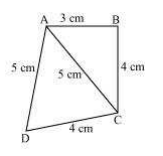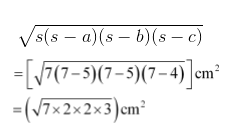Guru

# Find the area of a quadrilateral ABCD in which AB = 3 cm, BC = 4 cm, CD = 4 cm, DA = 5 cm and AC = 5 cm. Q.2

• 0

I want to know the best answer of the question from Heron’s Formula chapter of class 9th ncert math. The question from exercise 12.2  of math. Give me the easy way for solving this question of 2  Find the area of a quadrilateral ABCD in which AB = 3 cm, BC = 4 cm, CD = 4 cm, DA = 5 cm and AC = 5 cm.

Share

1. First, construct a diagram with the given parameter.Now, apply Pythagorean theorem in ΔABC,

AC2 = AB2+BC2

⇒ 52 = 32+42

⇒ 25 = 25

Thus, it can be concluded that ΔABC is a right angled at B.

So, area of ΔBCD = (½ ×3×4) = 6 cm2

The semi perimeter of ΔACD (s) = (perimeter/2) = (5+5+4)/2 cm = 14/2 cm = 7 m

Now, using Heron’s formula,

Area of ΔABD= 2√21 cm2 = 9.17 cm2 (approximately)

Area of quadrilateral ABCD = Area of ΔABC + Area of ΔABD = 6 cm2 +9.17 cm2 = 15.17 cm2

• 0